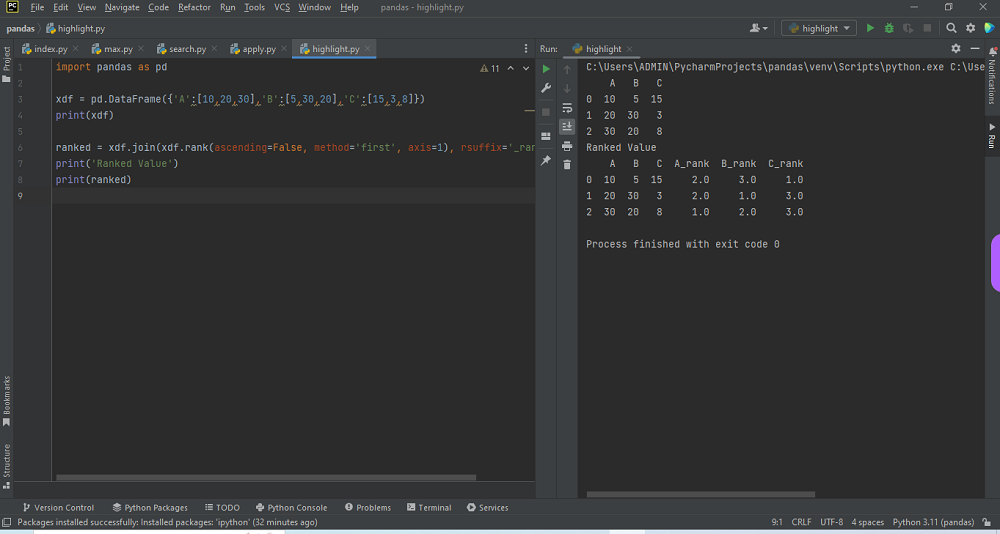# Python dataframe rank each column based on row valuesby vsasikalabeUpdated: Feb 28, 2023Solution Kit

Ranking each column based on row values in a Pandas DataFrame involves assigning a rank to each value based on its position among the values in that column. This can be useful in various scenarios, such as identifying the top or bottom values in a particular column, identifying outliers or anomalies in the data, or comparing the relative positions of different columns in the DataFrame.

In the code you provided, the rank() method is used to perform this ranking operation on each column of the DataFrame. The ascending=False parameter specifies that the ranks should be assigned in descending order (i.e., the highest values receive the lowest rank), and the method='first' parameter specifies that in cases where there are ties in the values, the rank should be assigned based on the order in which the values appear in the DataFrame.

The rank() method in Pandas calculates each element's rank in a DataFrame or a Series. By default, rank() calculates the average rank of each element in the group, but it also allows you to specify other methods to handle ties, such as min, max, and dense.

Here is some of the most common parameters for the rank() method:

• axis: specifies whether to calculate the rank across rows (axis=0, default) or columns (axis=1).
• method: specifies how to handle ties, with options including average, min, max, and dense.
• ascending: specifies whether to rank in ascending order (True, default) or descending order (False).

The resulting DataFrame includes the original columns, along with their corresponding ranks. This information can be used for further analysis or visualization, such as creating plots to compare the relative positions of different columns or identifying which columns contain the highest or lowest values. Ranking each column based on row values is a useful technique in data analysis. It can provide insights into the distribution and patterns in the data that might not be apparent from just looking at the raw values.

Here is an example of how to pandas dataframe ranks each column based on row values:Preview of the output that you will get on running this code from your IDE.

### Code

In this solution we used pandas library of python.

### Instructions

Follow the steps carefully to get the output easily.

2. Copy the snippet using the 'copy' and paste it in your IDE.
3. Add required dependencies and import them in Python file.
4. Run the file to generate the output.

I hope you found this useful. I have added the link to dependent libraries, version information in the following sections.

I found this code snippet by searching for ' Python dataframe rank each column based on row values 'in kandi. You can try any such use case!

### Environment Tested

I tested this solution in the following versions. Be mindful of changes when working with other versions.

1. The solution is created in PyCharm 2022.3.
2. The solution is tested on Python 3.11.1
3. Pandas version-1.5.2.

Using this solution, we are able to do Python dataframe rank each column based on row values with simple steps. This process also facilities an easy way to use, hassle-free method to create a hands-on working version of code which would help us to do Python dataframe rank each column based on row values.

### Dependent Library

pandasby pandas-dev

Python37363Version:v2.0.0rc1Flexible and powerful data analysis / manipulation library for Python, providing labeled data structures similar to R data.frame objects, statistical functions, and much more

Support
Quality
Security
Reuse

pandasby pandas-dev

Python37363Version:v2.0.0rc1License: Permissive (BSD-3-Clause)

Flexible and powerful data analysis / manipulation library for Python, providing labeled data structures similar to R data.frame objects, statistical functions, and much more
Support
Quality
Security